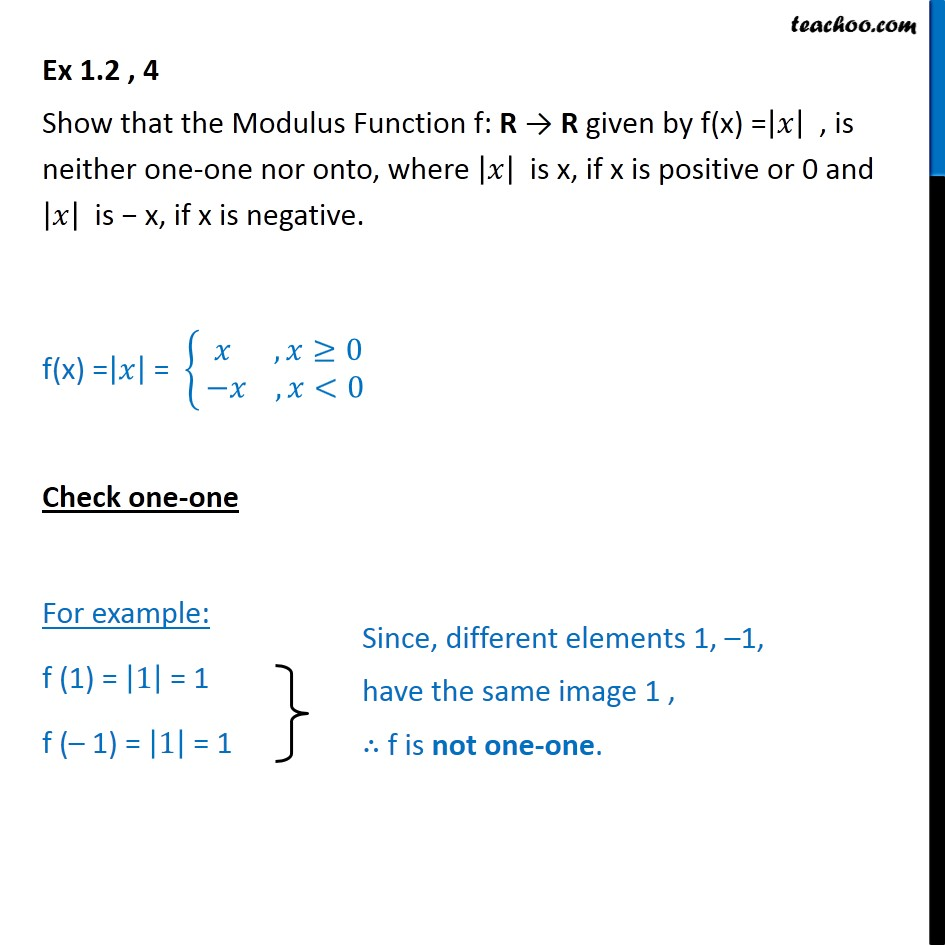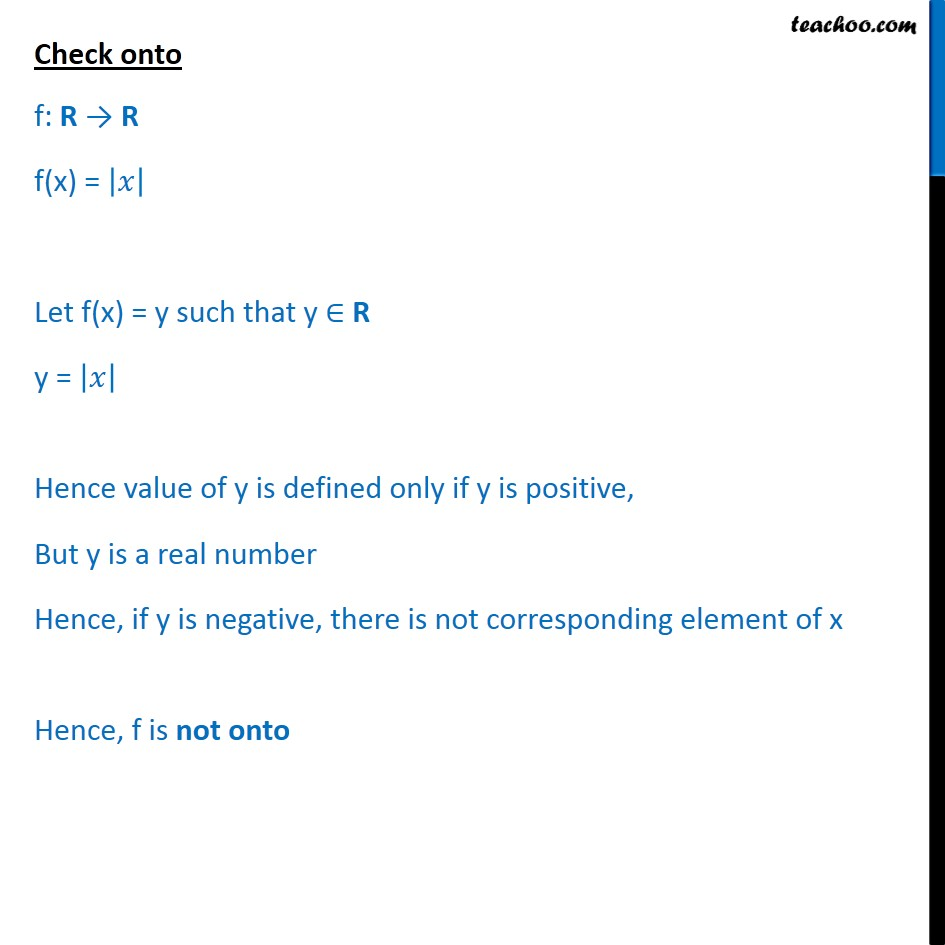1. Chapter 1 Class 12 Relation and Functions
2. Serial order wise
3. Ex 1.2

Transcript

Ex 1.2 , 4 Show that the Modulus Function f: R R given by f(x) = , is neither one-one nor onto, where is x, if x is positive or 0 and is x, if x is negative. f(x) = = , 0 , <0 Check one-one For example: f (1) = 1 = 1 f ( 1) = 1 = 1 Check onto f: R R f(x) = Let f(x) = y such that y R y = Hence value of y is defined only if y is positive, But y is a real number Hence, if y is negative, there is not corresponding element of x Hence, f is not onto

Ex 1.2

About the AuthorDavneet Singh
Davneet Singh is a graduate from Indian Institute of Technology, Kanpur. He has been teaching from the past 9 years. He provides courses for Maths and Science at Teachoo.